# snssde1d()

Assume that we want to describe the following SDE:

It? form3:

$\begin{equation}\label{eq:05} dX_{t} = \frac{1}{2}\theta^{2} X_{t} dt + \theta X_{t} dW_{t},\qquad X_{0}=x_{0} > 0 \end{equation}$

Stratonovich form: $\begin{equation}\label{eq:06} dX_{t} = \frac{1}{2}\theta^{2} X_{t} dt +\theta X_{t} \circ dW_{t},\qquad X_{0}=x_{0} > 0 \end{equation}$

In the above $$f(t,x)=\frac{1}{2}\theta^{2} x$$ and $$g(t,x)= \theta x$$ ($$\theta > 0$$), $$W_{t}$$ is a standard Wiener process. To simulate this models using snssde1d() function we need to specify:

• The drift and diffusion coefficients as R expressions that depend on the state variable x and time variable t.
• The number of simulation steps N=1000 (by default: N=1000).
• The number of the solution trajectories to be simulated by M=1000 (by default: M=1).
• The initial conditions t0=0, x0=10 and end time T=1 (by default: t0=0, x0=0 and T=1).
• The integration step size Dt=0.001 (by default: Dt=(T-t0)/N).
• The choice of process types by the argument type="ito" for Ito or type="str" for Stratonovich (by default type="ito").
• The numerical method to be used by method (by default method="euler").
R> theta = 0.5
R> f <- expression( (0.5*theta^2*x) )
R> g <- expression( theta*x )
R> mod1 <- snssde1d(drift=f,diffusion=g,x0=10,M=1000,type="ito") # Using It?
R> mod2 <- snssde1d(drift=f,diffusion=g,x0=10,M=1000,type="str") # Using Stratonovich
R> mod1
Itô Sde 1D:
| dX(t) = (0.5 * theta^2 * X(t)) * dt + theta * X(t) * dW(t)
Method:
| Euler scheme with order 0.5
Summary:
| Size of process   | N  = 1001.
| Number of simulation  | M  = 1000.
| Initial value     | x0 = 10.
| Time of process   | t in [0,1].
| Discretization    | Dt = 0.001.
R> mod2
Stratonovich Sde 1D:
| dX(t) = (0.5 * theta^2 * X(t)) * dt + theta * X(t) o dW(t)
Method:
| Euler scheme with order 0.5
Summary:
| Size of process   | N  = 1001.
| Number of simulation  | M  = 1000.
| Initial value     | x0 = 10.
| Time of process   | t in [0,1].
| Discretization    | Dt = 0.001.

Using Monte-Carlo simulations, the following statistical measures (S3 method) for class snssde1d() can be approximated for the $$X_{t}$$ process at any time $$t$$:

• The expected value $$\text{E}(X_{t})$$ at time $$t$$, using the command mean.
• The variance $$\text{Var}(X_{t})$$ at time $$t$$, using the command moment with order=2 and center=TRUE.
• The median $$\text{Med}(X_{t})$$ at time $$t$$, using the command Median.
• The mode $$\text{Mod}(X_{t})$$ at time $$t$$, using the command Mode.
• The quartile of $$X_{t}$$ at time $$t$$, using the command quantile.
• The maximum and minimum of $$X_{t}$$ at time $$t$$, using the command min and max.
• The skewness and the kurtosis of $$X_{t}$$ at time $$t$$, using the command skewness and kurtosis.
• The coefficient of variation (relative variability) of $$X_{t}$$ at time $$t$$, using the command cv.
• The central moments up to order $$p$$ of $$X_{t}$$ at time $$t$$, using the command moment.
• The empirical $$\alpha \%$$ confidence interval of expected value $$\text{E}(X_{t})$$ at time $$t$$ (from the $$2.5th$$ to the $$97.5th$$ percentile), using the command bconfint.
• The result summaries of the results of Monte-Carlo simulation at time $$t$$, using the command summary.

The following statistical measures (S3 method) for class snssde1d() can be approximated for the $$X_{t}$$ process at any time $$t$$, for example at=1:

R> s = 1
R> mean(mod1, at = s)
 11.141
R> moment(mod1, at = s , center = TRUE , order = 2) ## variance
 31.678
R> Median(mod1, at = s)
 9.9339
R> Mode(mod1, at =s)
 7.8949
R> quantile(mod1 , at = s)
     0%     25%     50%     75%    100%
1.7029  6.9402  9.9339 14.1501 40.1214 
R> kurtosis(mod1 , at = s)
 5.049
R> skewness(mod1 , at = s)
 1.2197
R> cv(mod1 , at = s )
 0.50546
R> min(mod1 , at = s)
 1.7029
R> max(mod1 , at = s)
 40.121
R> moment(mod1, at = s , center= TRUE , order = 4)
 5076.7
R> moment(mod1, at = s , center= FALSE , order = 4)
 53776

The summary of the results of mod1 and mod2 at time $$t=1$$ of class snssde1d() is given by:

R> summary(mod1, at = 1)

Monte-Carlo Statistics for X(t) at time t = 1

Mean                  11.14059
Variance              31.70956
Median                 9.93394
Mode                   7.89493
First quartile         6.94024
Third quartile        14.15014
Minimum                1.70286
Maximum               40.12143
Skewness               1.21974
Kurtosis               5.04898
Coef-variation         0.50546
3th-order moment     217.79819
4th-order moment    5076.73338
5th-order moment   88870.00801
6th-order moment 1991341.09731
R> summary(mod2, at = 1)

Monte-Carlo Statistics for X(t) at time t = 1

Mean                   9.79629
Variance              30.56349
Median                 8.62333
Mode                   6.37665
First quartile         5.98119
Third quartile        11.98768
Minimum                1.86562
Maximum               41.72127
Skewness               1.78504
Kurtosis               7.96468
Coef-variation         0.56434
3th-order moment     301.61461
4th-order moment    7440.02410
5th-order moment  171076.23474
6th-order moment 4520033.64638

Hence we can just make use of the rsde1d() function to build our random number generator for the conditional density of the $$X_{t}|X_{0}$$ ($$X_{t}^{\text{mod1}}| X_{0}$$ and $$X_{t}^{\text{mod2}}|X_{0}$$) at time $$t = 1$$.

R> x1 <- rsde1d(object = mod1, at = 1)  # X(t=1) | X(0)=x0 (It? SDE)
R> x2 <- rsde1d(object = mod2, at = 1)  # X(t=1) | X(0)=x0 (Stratonovich SDE)
R> head(x1,n=10)
   7.8535  9.7246 21.2346  8.3660 12.9236 14.8307  9.7619 12.7098
 17.6658 14.5872
R> head(x2,n=10)
   7.9676 10.0006  9.8624  5.4222  9.1462  8.5774 19.4367 10.6745
  7.3757 15.1997
R> summary(data.frame(x1,x2))
       x1              x2
Min.   : 1.70   Min.   : 1.87
1st Qu.: 6.94   1st Qu.: 5.98
Median : 9.93   Median : 8.62
Mean   :11.14   Mean   : 9.80
3rd Qu.:14.15   3rd Qu.:11.99
Max.   :40.12   Max.   :41.72  

The function dsde1d() can be used to show the Approximate transitional density for $$X_{t}|X_{0}$$ at time $$t-s=1$$ with log-normal curves:

R> mu1 = log(10); sigma1= sqrt(theta^2)  # log mean and log variance for mod1
R> mu2 = log(10)-0.5*theta^2 ; sigma2 = sqrt(theta^2) # log mean and log variance for mod2
R> AppdensI <- dsde1d(mod1, at = 1)
R> AppdensS <- dsde1d(mod2, at = 1)
R> plot(AppdensI , dens = function(x) dlnorm(x,meanlog=mu1,sdlog = sigma1))
R> plot(AppdensS , dens = function(x) dlnorm(x,meanlog=mu2,sdlog = sigma2))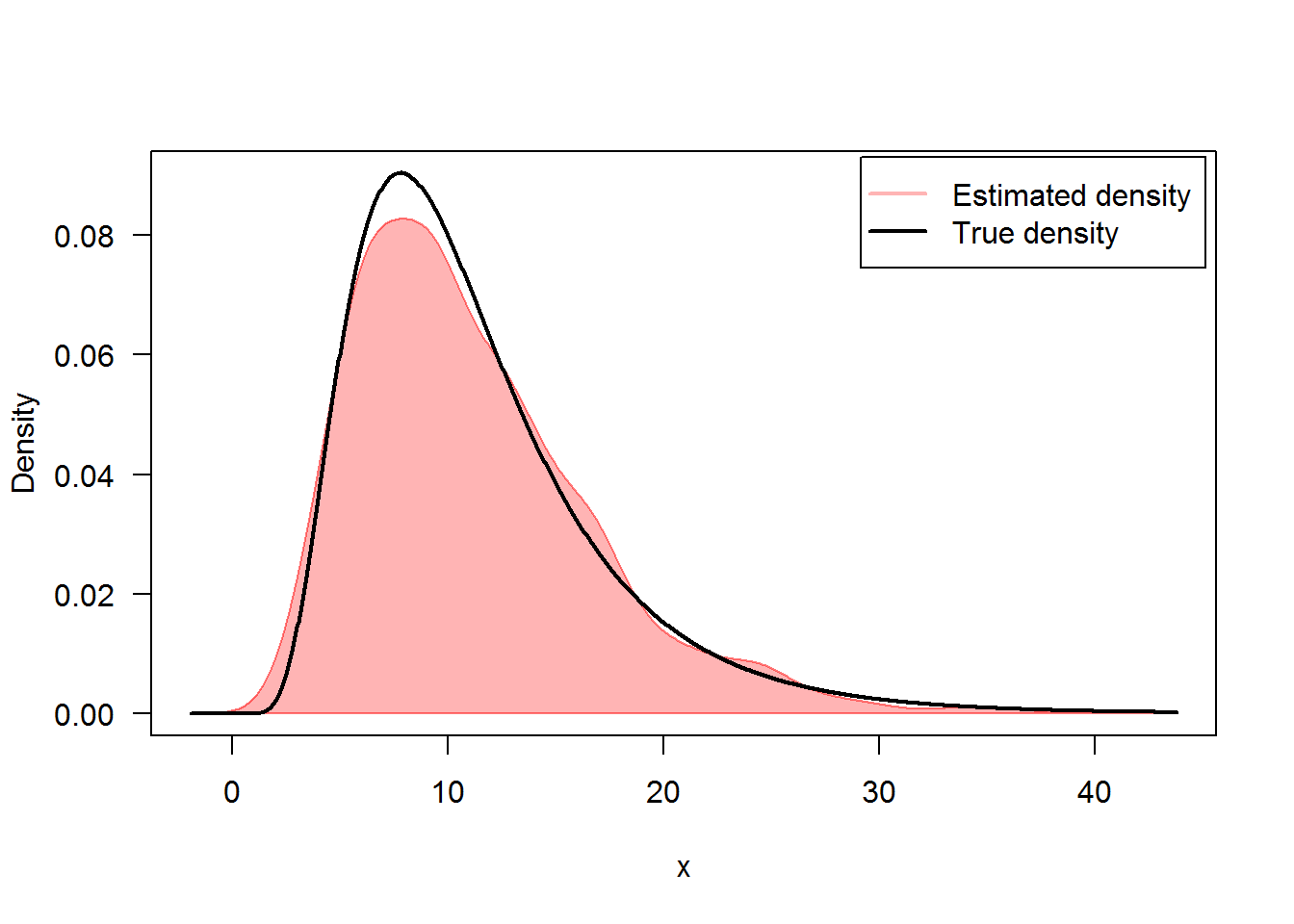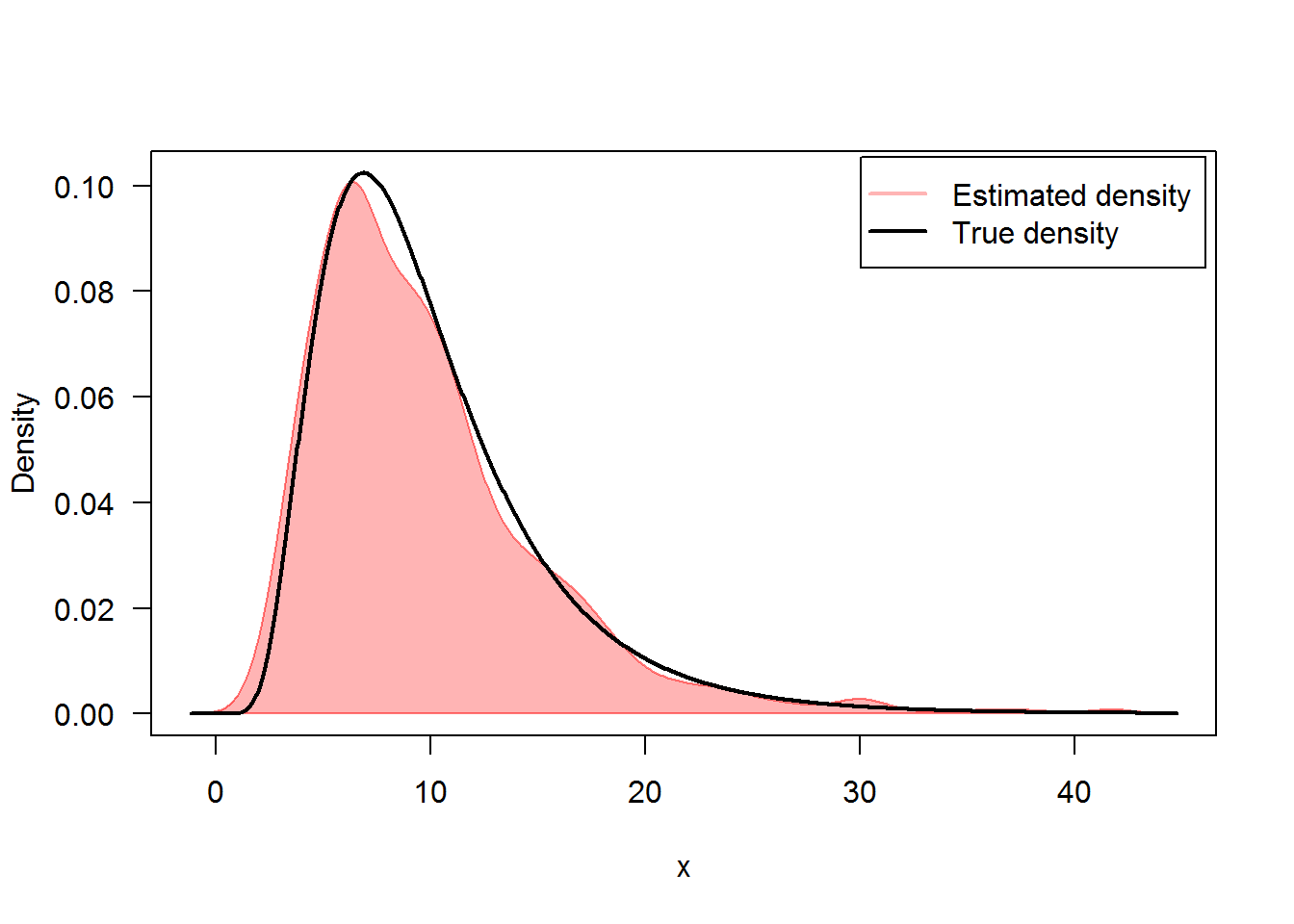In Figure 2, we present the flow of trajectories, the mean path (red lines) of solution of and , with their empirical $$95\%$$ confidence bands, that is to say from the $$2.5th$$ to the $$97.5th$$ percentile for each observation at time $$t$$ (blue lines):

R> ## It?
R> plot(mod1,ylab=expression(X^mod1))
R> lines(time(mod1),apply(mod1$X,1,mean),col=2,lwd=2) R> lines(time(mod1),apply(mod1$X,1,bconfint,level=0.95)[1,],col=4,lwd=2)
R> lines(time(mod1),apply(mod1$X,1,bconfint,level=0.95)[2,],col=4,lwd=2) R> legend("topleft",c("mean path",paste("bound of", 95,"% confidence")),inset = .01,col=c(2,4),lwd=2,cex=0.8) R> ## Stratonovich R> plot(mod2,ylab=expression(X^mod2)) R> lines(time(mod2),apply(mod2$X,1,mean),col=2,lwd=2)
R> lines(time(mod2),apply(mod2$X,1,bconfint,level=0.95)[1,],col=4,lwd=2) R> lines(time(mod2),apply(mod2$X,1,bconfint,level=0.95)[2,],col=4,lwd=2)
R> legend("topleft",c("mean path",paste("bound of",95,"% confidence")),col=c(2,4),inset =.01,lwd=2,cex=0.8)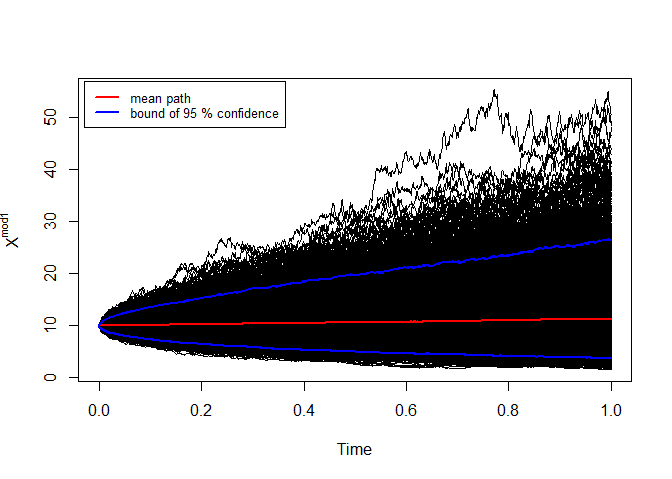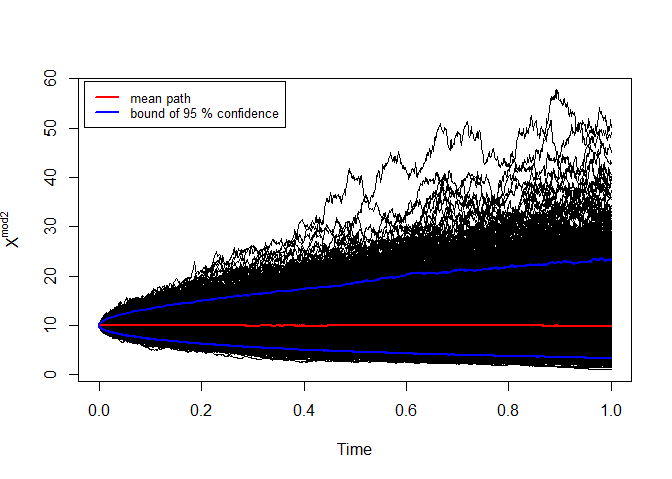mod1: It? and mod2: Stratonovich

Return to snssde1d()

# snssde2d()

The following $$2$$-dimensional SDE’s with a vector of drift and a diagonal matrix of diffusion coefficients:

It? form: $\begin{equation}\label{eq:09} \begin{cases} dX_t = f_{x}(t,X_{t},Y_{t}) dt + g_{x}(t,X_{t},Y_{t}) dW_{1,t}\\ dY_t = f_{y}(t,X_{t},Y_{t}) dt + g_{y}(t,X_{t},Y_{t}) dW_{2,t} \end{cases} \end{equation}$

Stratonovich form: $\begin{equation}\label{eq:10} \begin{cases} dX_t = f_{x}(t,X_{t},Y_{t}) dt + g_{x}(t,X_{t},Y_{t}) \circ dW_{1,t}\\ dY_t = f_{y}(t,X_{t},Y_{t}) dt + g_{y}(t,X_{t},Y_{t}) \circ dW_{2,t} \end{cases} \end{equation}$ $$W_{1,t}$$ and $$W_{2,t}$$ is a two independent standard Wiener process. To simulate $$2d$$ models using snssde2d() function we need to specify:

• The drift (2d) and diffusion (2d) coefficients as R expressions that depend on the state variable x, y and time variable t.
• The number of simulation steps N (default: N=1000).
• The number of the solution trajectories to be simulated by M (default: M=1).
• The initial conditions t0, x0 and end time T (default: t0=0, x0=c(0,0) and T=1).
• The integration step size Dt (default: Dt=(T-t0)/N).
• The choice of process types by the argument type="ito" for Ito or type="str" for Stratonovich (default type="ito").
• The numerical method to be used by method (default method="euler").

## Ornstein-Uhlenbeck process and its integral

The Ornstein-Uhlenbeck (OU) process has a long history in physics. Introduced in essence by Langevin in his famous 1908 paper on Brownian motion, the process received a more thorough mathematical examination several decades later by Uhlenbeck and Ornstein (1930). The OU process is understood here to be the univariate continuous Markov process $$X_t$$. In mathematical terms, the equation is written as an Ito equation: $\begin{equation}\label{eq016} dX_t = -\frac{1}{\mu} X_t dt + \sqrt{\sigma} dW_t,\quad X_{0}=x_{0} \end{equation}$ In these equations, $$\mu$$ and $$\sigma$$ are positive constants called, respectively, the relaxation time and the diffusion constant. The time integral of the OU process $$X_t$$ (or indeed of any process $$X_t$$) is defined to be the process $$Y_t$$ that satisfies: $\begin{equation}\label{eq017} Y_{t} = Y_{0}+\int X_{t} dt \Leftrightarrow dY_t = X_{t} dt ,\quad Y_{0}=y_{0} \end{equation}$ $$Y_t$$ is not itself a Markov process; however, $$X_t$$ and $$Y_t$$ together comprise a bivariate continuous Markov process. We wish to find the solutions $$X_t$$ and $$Y_t$$ to the coupled time-evolution equations: $\begin{equation}\label{eq018} \begin{cases} dX_t = -\frac{1}{\mu} X_t dt + \sqrt{\sigma} dW_t\\ dY_t = X_{t} dt \end{cases} \end{equation}$

We simulate a flow of $$1000$$ trajectories of $$(X_{t},Y_{t})$$, with integration step size $$\Delta t = 0.01$$, and using second Milstein method.

R> x0=5;y0=0
R> mu=3;sigma=0.5
R> fx <- expression(-(x/mu),x)
R> gx <- expression(sqrt(sigma),0)
R> mod2d <- snssde2d(drift=fx,diffusion=gx,Dt=0.01,M=1000,x0=c(x0,y0),method="smilstein")
R> mod2d
Itô Sde 2D:
| dX(t) = -(X(t)/mu) * dt + sqrt(sigma) * dW1(t)
| dY(t) = X(t) * dt + 0 * dW2(t)
Method:
| Second-order Milstein scheme
Summary:
| Size of process   | N  = 1001.
| Number of simulation  | M  = 1000.
| Initial values    | (x0,y0) = (5,0).
| Time of process   | t in [0,10].
| Discretization    | Dt = 0.01.

The following statistical measures (S3 method) for class snssde2d() can be approximated for the $$(X_{t},Y_{t})$$ process at any time $$t$$, for example at=5:

R> s = 5
R> mean(mod2d, at = s)
  0.92679 12.21988
R> moment(mod2d, at = s , center = TRUE , order = 2) ## variance
 0.68889 6.66566
R> Median(mod2d, at = s)
  0.93172 12.17180
R> Mode(mod2d, at = s)
  1.0114 12.2733
R> quantile(mod2d , at = s)
$x 0% 25% 50% 75% 100% -1.76975 0.32673 0.93172 1.47083 3.93978$y
0%     25%     50%     75%    100%
1.1376 10.4418 12.1718 13.9680 20.7411 
R> kurtosis(mod2d , at = s)
 3.0766 3.2350
R> skewness(mod2d , at = s)
 0.040533 0.055974
R> cv(mod2d , at = s )
 0.89601 0.21138
R> min(mod2d , at = s)
 -1.7698  1.1376
R> max(mod2d , at = s)
  3.9398 20.7411
R> moment(mod2d, at = s , center= TRUE , order = 4)
   1.463 144.020
R> moment(mod2d, at = s , center= FALSE , order = 4)
     5.837 28461.365

The summary of the results of mod2d at time $$t=5$$ of class snssde2d() is given by:

R> summary(mod2d, at = s)

Monte-Carlo Statistics for (X(t),Y(t)) at time t = 5
X          Y
Mean              0.92679   12.21988
Variance          0.68958    6.67233
Median            0.93172   12.17180
Mode              1.01144   12.27334
First quartile    0.32673   10.44181
Third quartile    1.47083   13.96801
Minimum          -1.76975    1.13758
Maximum           3.93978   20.74114
Skewness          0.04053    0.05597
Kurtosis          3.07656    3.23495
Coef-variation    0.89601    0.21138
3th-order moment  0.02321    0.96472
4th-order moment  1.46296  144.02011
5th-order moment  0.09333  -55.09065
6th-order moment  5.28267 6095.24983

For plotting (back in time) using the command plot, the results of the simulation are shown in Figure 3.

R> plot(mod2d)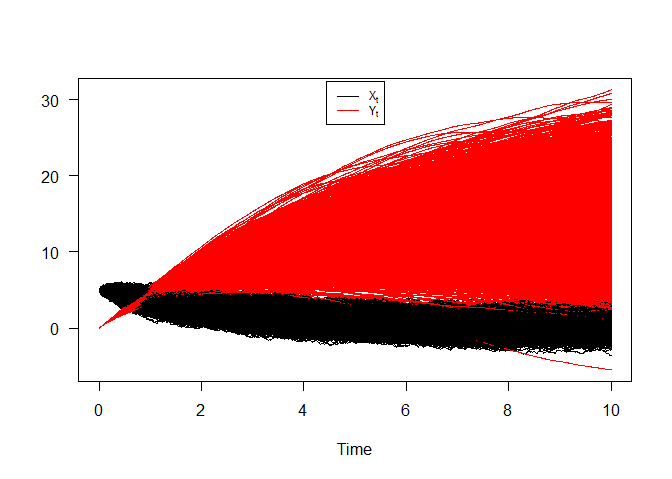Ornstein-Uhlenbeck process and its integral

Take note of the well known result, which can be derived from either this equations. That for any $$t > 0$$ the OU process $$X_t$$ and its integral $$Y_t$$ will be the normal distribution with mean and variance given by: $\begin{cases} \text{E}(X_{t}) =x_{0} e^{-t/\mu} &\text{and}\quad\text{Var}(X_{t})=\frac{\sigma \mu}{2} \left (1-e^{-2t/\mu}\right )\\ \text{E}(Y_{t}) = y_{0}+x_{0}\mu \left (1-e^{-t/\mu}\right ) &\text{and}\quad\text{Var}(Y_{t})=\sigma\mu^{3}\left (\frac{t}{\mu}-2\left (1-e^{-t/\mu}\right )+\frac{1}{2}\left (1-e^{-2t/\mu}\right )\right ) \end{cases}$

Hence we can just make use of the rsde2d() function to build our random number for $$(X_{t},Y_{t})$$ at time $$t = 10$$.

R> out <- rsde2d(object = mod2d, at = 10)
R> head(out,n=10)
          x       y
1  -0.40797 20.2867
2  -0.19133 12.1643
3  -0.75371  9.4854
4   0.30984 13.3197
5  -0.87097 13.6577
6   1.29402 16.1173
7   0.63369 13.9212
8   1.37924 18.2539
9  -0.44067 20.0510
10 -1.38257  3.5238
R> summary(out)
       x                y
Min.   :-3.102   Min.   :-2.02
1st Qu.:-0.488   1st Qu.:11.03
Median : 0.168   Median :13.91
Mean   : 0.151   Mean   :14.27
3rd Qu.: 0.765   3rd Qu.:17.75
Max.   : 2.860   Max.   :33.57  
R> cov(out)
       x       y
x 0.8335  2.2577
y 2.2577 25.4355

Figure 4, show simulation results for moments of system :

R> mx <- apply(mod2d$X,1,mean) R> my <- apply(mod2d$Y,1,mean)
R> Sx <- apply(mod2d$X,1,var) R> Sy <- apply(mod2d$Y,1,var)
R> Cxy <- sapply(1:1001,function(i) cov(mod2d$X[i,],mod2d$Y[i,]))
R> out_b <- data.frame(mx,my,Sx,Sy,Cxy)
R> matplot(time(mod2d), out_b, type = "l", xlab = "time", ylab = "",col=2:6,lwd=2,lty=2:6,las=1)
R> legend("topleft",c(expression(hat(E)(X[t]),hat(E)(Y[t]),hat(Var)(X[t]),hat(Var)(Y[t]),hat(Cov)(X[t],Y[t]))),inset = .05,col=2:6,lty=2:6,lwd=2,cex=0.9)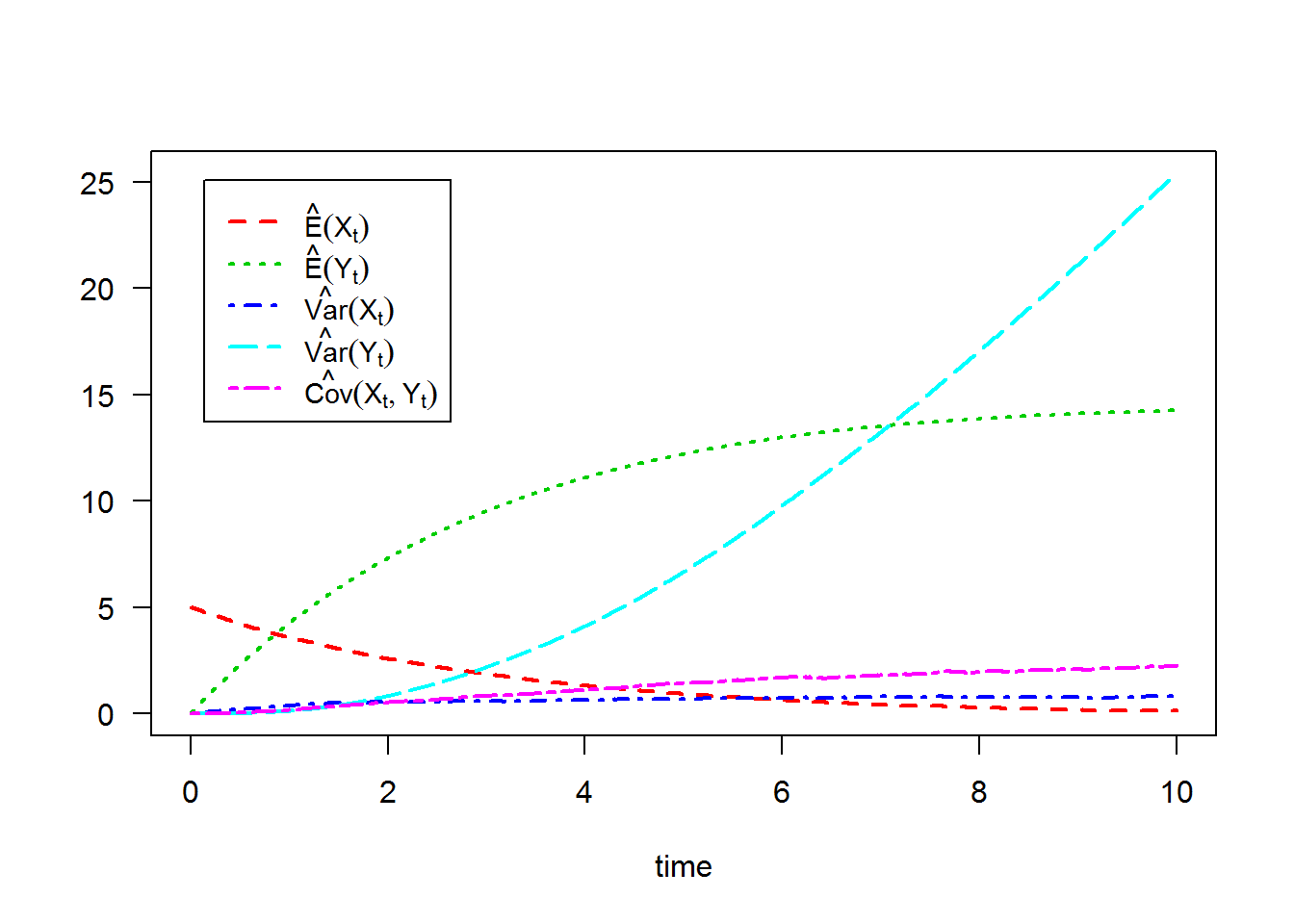For each SDE type and for each numerical scheme, the density of $$X_t$$ and $$Y_t$$ at time $$t=10$$ are reported using dsde2d() function, see e.g. Figure 5: the marginal density of $$X_t$$ and $$Y_t$$ at time $$t=10$$.

R> denM <- dsde2d(mod2d,pdf="M",at =10)
R> denM

Marginal density of X(t-t0)|X(t0)=5 at time t = 10

Data: x (1000 obs.);    Bandwidth 'bw' = 0.2064

x                f(x)
Min.   :-3.7208   Min.   :0.00002
1st Qu.:-1.9207   1st Qu.:0.00727
Median :-0.1207   Median :0.06398
Mean   :-0.1207   Mean   :0.13875
3rd Qu.: 1.6794   3rd Qu.:0.27940
Max.   : 3.4794   Max.   :0.42392

Marginal density of Y(t-t0)|Y(t0)=0 at time t = 10

Data: y (1000 obs.);    Bandwidth 'bw' = 1.133

y               f(y)
Min.   :-5.422   Min.   :0.000004
1st Qu.: 5.177   1st Qu.:0.000781
Median :15.776   Median :0.008865
Mean   :15.776   Mean   :0.023565
3rd Qu.:26.375   3rd Qu.:0.046913
Max.   :36.973   Max.   :0.084134  
R> plot(denM, main="Marginal Density")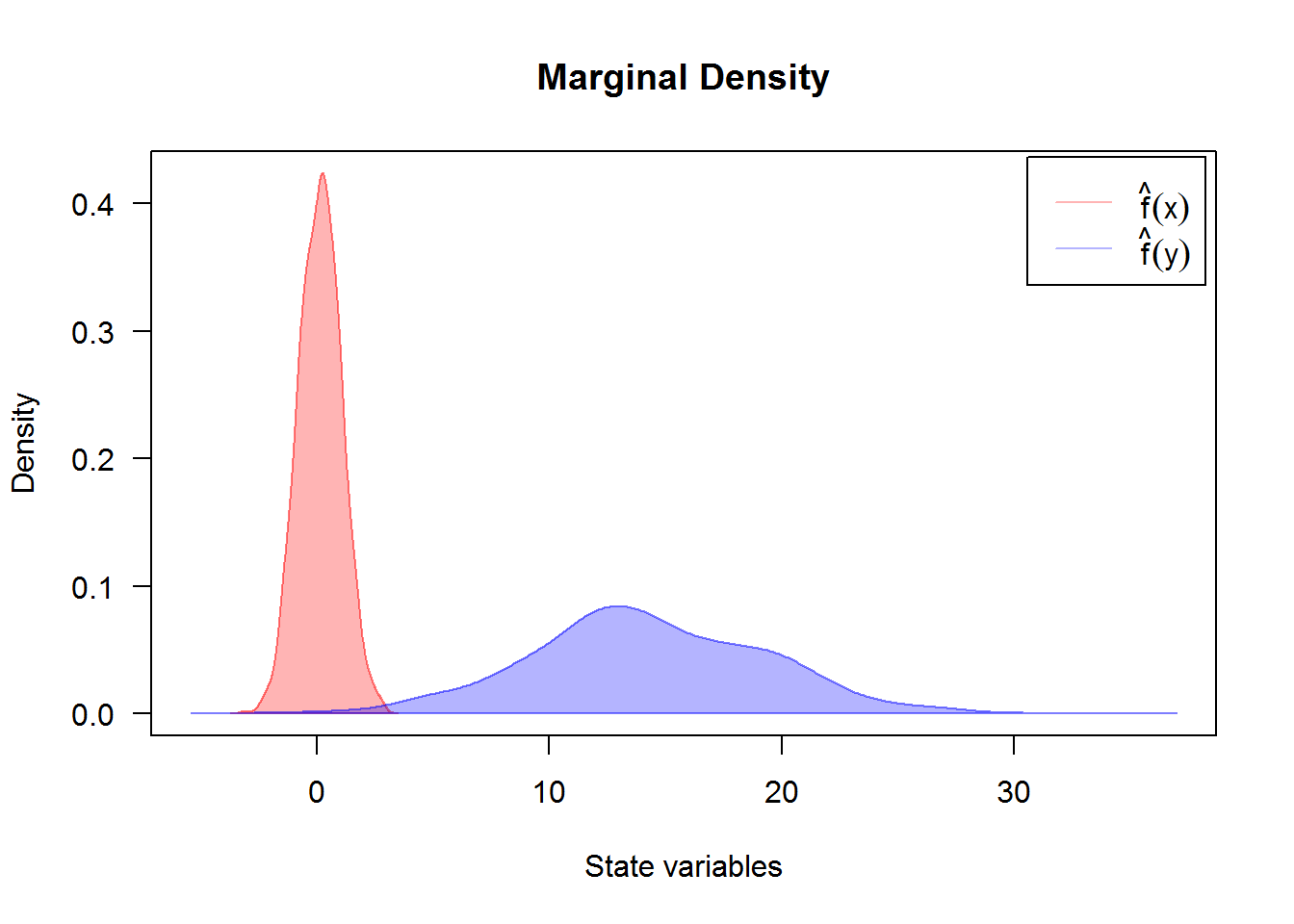Created using dsde2d() plotted in (x, y)-space with dim = 2. A contour and image plot of density obtained from a realization of system $$(X_{t},Y_{t})$$ at time t=10.

R> denJ <- dsde2d(mod2d, pdf="J", n=100,at =10)
R> denJ

Joint density of (X(t-t0),Y(t-t0)|X(t0)=5,Y(t0)=0) at time t = 10

Data: (x,y) (2 x 1000 obs.);

x                  y              f(x,y)
Min.   :-3.10162   Min.   :-2.022   Min.   :0.000000
1st Qu.:-1.61115   1st Qu.: 6.877   1st Qu.:0.000028
Median :-0.12068   Median :15.776   Median :0.000690
Mean   :-0.12068   Mean   :15.776   Mean   :0.004603
3rd Qu.: 1.36980   3rd Qu.:24.675   3rd Qu.:0.004779
Max.   : 2.86027   Max.   :33.574   Max.   :0.037396  
R> plot(denJ,display="contour",main="Bivariate Transition Density at time t=10")
R> plot(denJ,display="image",main="Bivariate Transition Density at time t=10")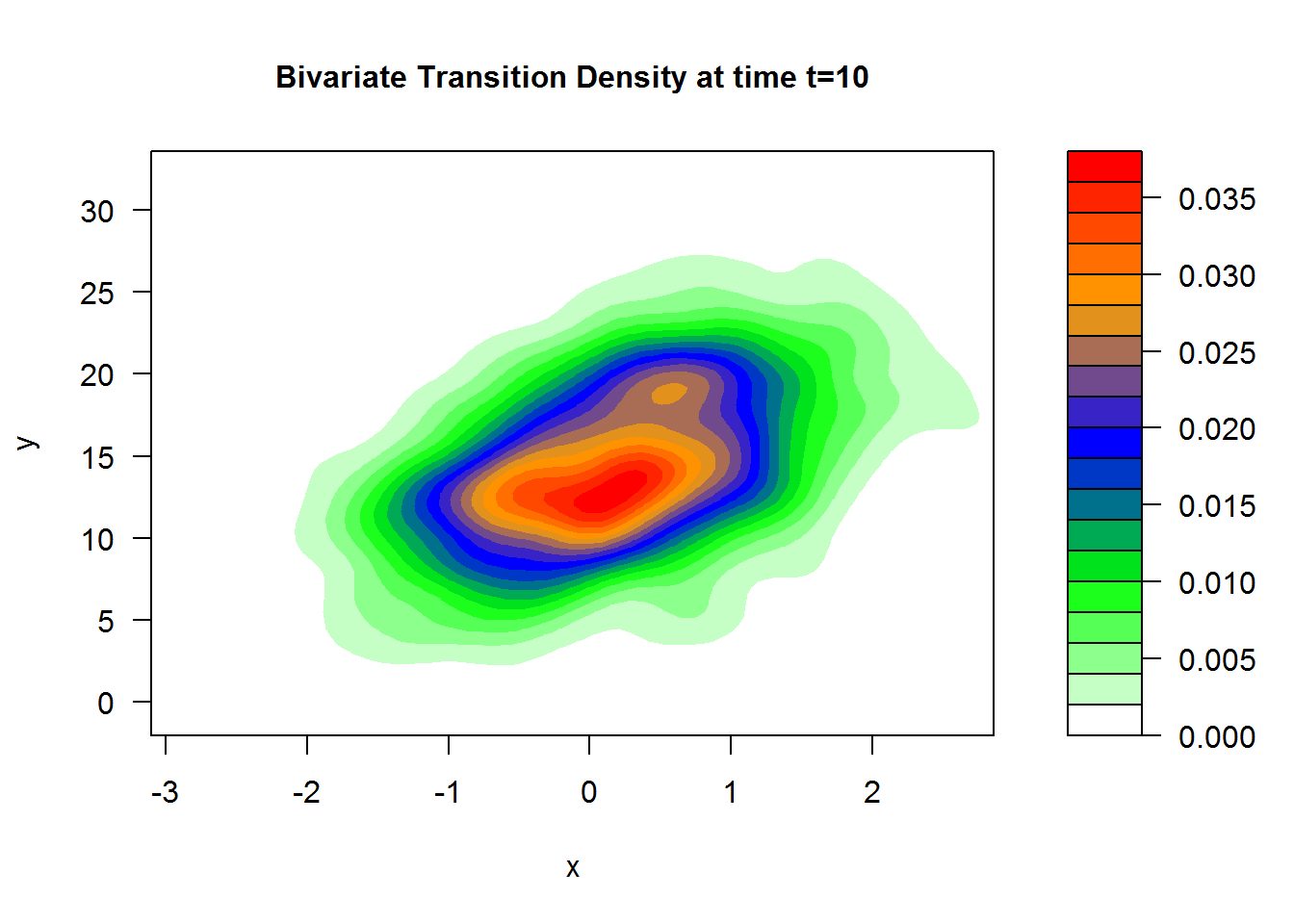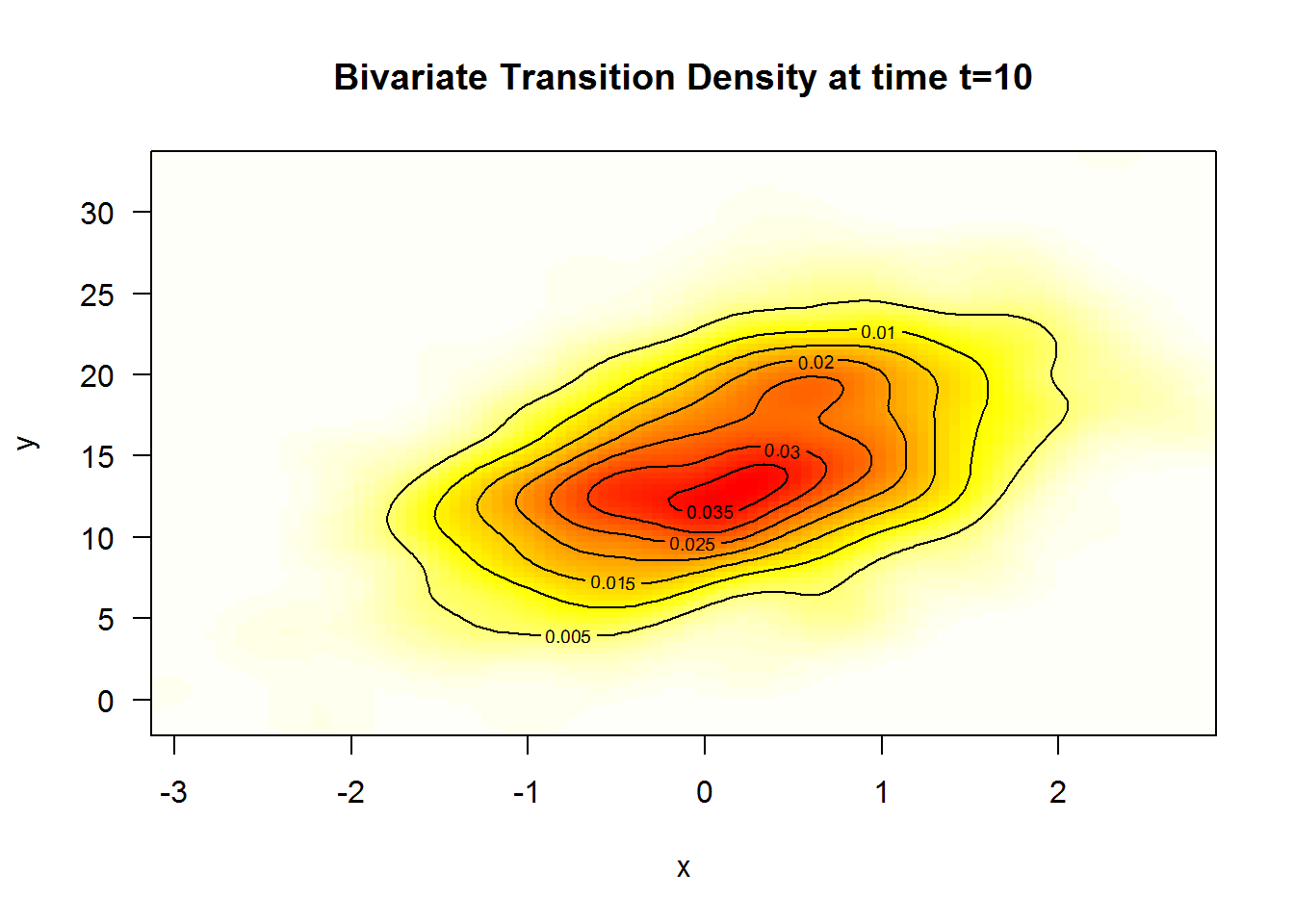A $$3$$D plot of the transition density at $$t=10$$ obtained with:

R> plot(denJ,main="Bivariate Transition Density at time t=10")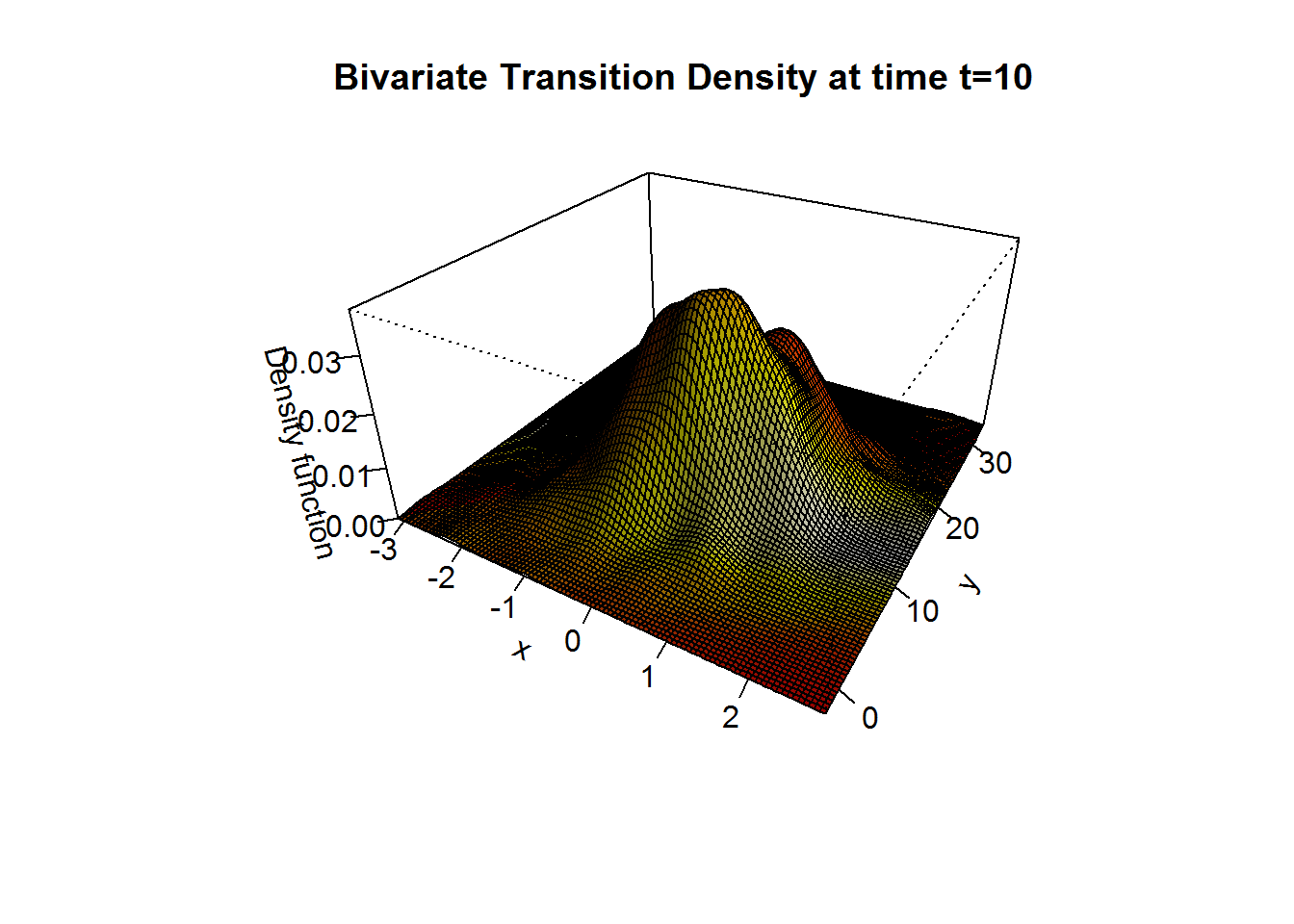We approximate the bivariate transition density over the set transition horizons $$t\in [1,10]$$ by $$\Delta t = 0.005$$ using the code:

R> for (i in seq(1,10,by=0.005)){
+ plot(dsde2d(mod2d, at = i,n=100),display="contour",main=paste0('Transition Density \n t = ',i))
+ }

Return to snssde2d()

## The stochastic Van-der-Pol equation

The Van der Pol (1922) equation is an ordinary differential equation that can be derived from the Rayleigh differential equation by differentiating and setting $$\dot{x}=y$$, see Naess and Hegstad (1994); Leung (1995) and for more complex dynamics in Van-der-Pol equation see Jing et al. (2006). It is an equation describing self-sustaining oscillations in which energy is fed into small oscillations and removed from large oscillations. This equation arises in the study of circuits containing vacuum tubes and is given by: $\begin{equation}\label{eq:12} \ddot{X}-\mu (1-X^{2}) \dot{X} + X = 0 \end{equation}$ where $$x$$ is the position coordinate (which is a function of the time $$t$$), and $$\mu$$ is a scalar parameter indicating the nonlinearity and the strength of the damping, to simulate the deterministic equation see Grayling (2014) for more details. Consider stochastic perturbations of the Van-der-Pol equation, and random excitation force of such systems by White noise $$\xi_{t}$$, with delta-type correlation function $$\text{E}(\xi_{t}\xi_{t+h})=2\sigma \delta (h)$$ $\begin{equation}\label{eq:13} \ddot{X}-\mu (1-X^{2}) \dot{X} + X = \xi_{t}, \end{equation}$ where $$\mu > 0$$ . It’s solution cannot be obtained in terms of elementary functions, even in the phase plane. The White noise $$\xi_{t}$$ is formally derivative of the Wiener process $$W_{t}$$. The representation of a system of two first order equations follows the same idea as in the deterministic case by letting $$\dot{x}=y$$, from physical equation we get the above system: $\begin{equation}\label{eq:14} \begin{cases} \dot{X} = Y \\ \dot{Y} = \mu \left(1-X^{2}\right) Y - X + \xi_{t} \end{cases} \end{equation}$ The system can mathematically explain by a Stratonovitch equations: $\begin{equation}\label{eq:15} \begin{cases} dX_{t} = Y_{t} dt \\ dY_{t} = \left(\mu (1-X^{2}_{t}) Y_{t} - X_{t}\right) dt + 2 \sigma \circ dW_{2,t} \end{cases} \end{equation}$

Implemente in R as follows, with integration step size $$\Delta t = 0.01$$ and using stochastic Runge-Kutta methods 1-stage.

R> mu = 4; sigma=0.1
R> fx <- expression( y ,  (mu*( 1-x^2 )* y - x))
R> gx <- expression( 0 ,2*sigma)
R> mod2d <- snssde2d(drift=fx,diffusion=gx,N=10000,Dt=0.01,type="str",method="rk1")
R> mod2d
Stratonovich Sde 2D:
| dX(t) = Y(t) * dt + 0 o dW1(t)
| dY(t) = (mu * (1 - X(t)^2) * Y(t) - X(t)) * dt + 2 * sigma o dW2(t)
Method:
| Runge-Kutta method with order 1
Summary:
| Size of process   | N  = 10001.
| Number of simulation  | M  = 1.
| Initial values    | (x0,y0) = (0,0).
| Time of process   | t in [0,100].
| Discretization    | Dt = 0.01.

For plotting (back in time) using the command plot, and plot2d in plane the results of the simulation are shown in Figure 8.

R> plot2d(mod2d) ## in plane (O,X,Y)
R> plot(mod2d)   ## back in time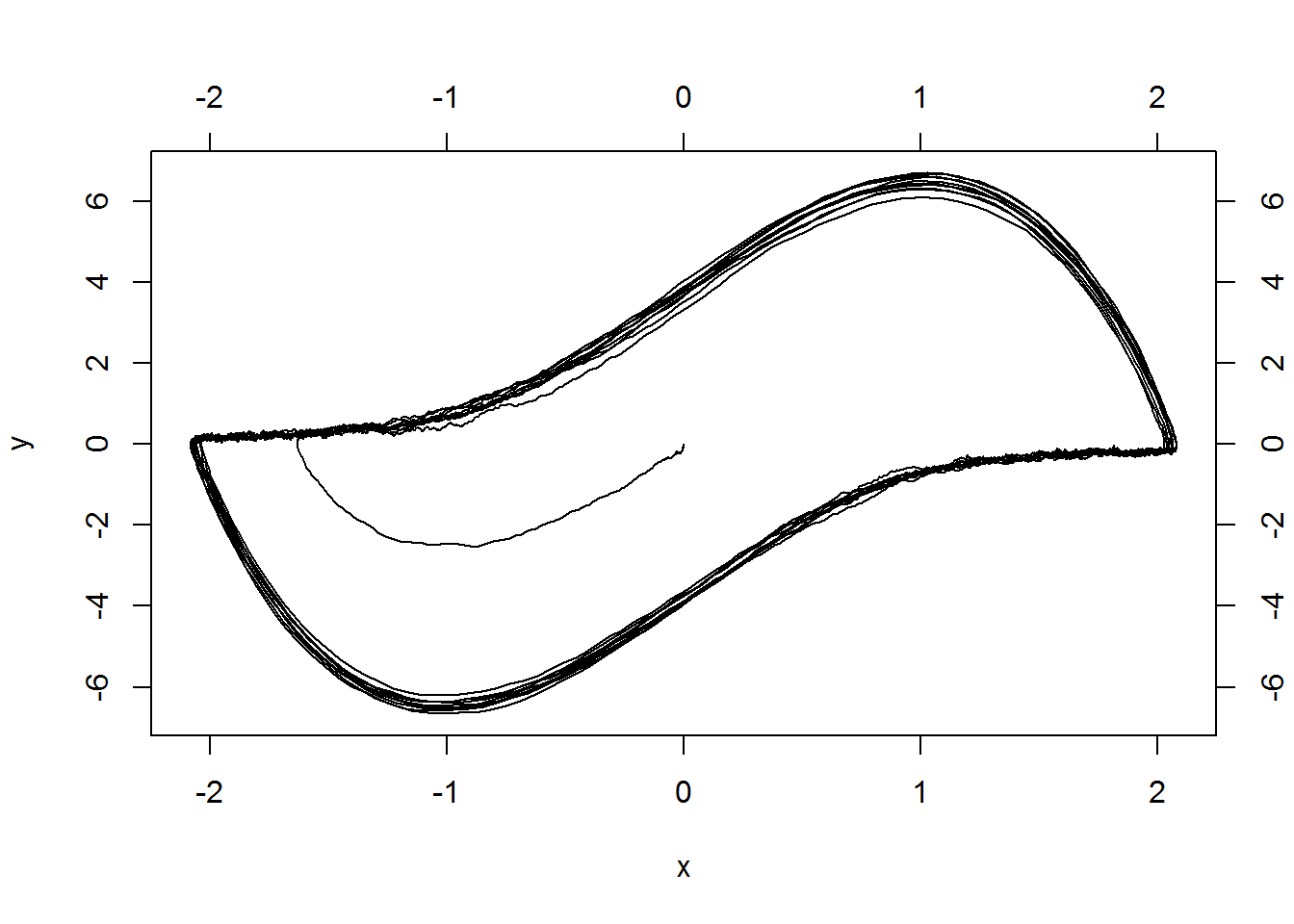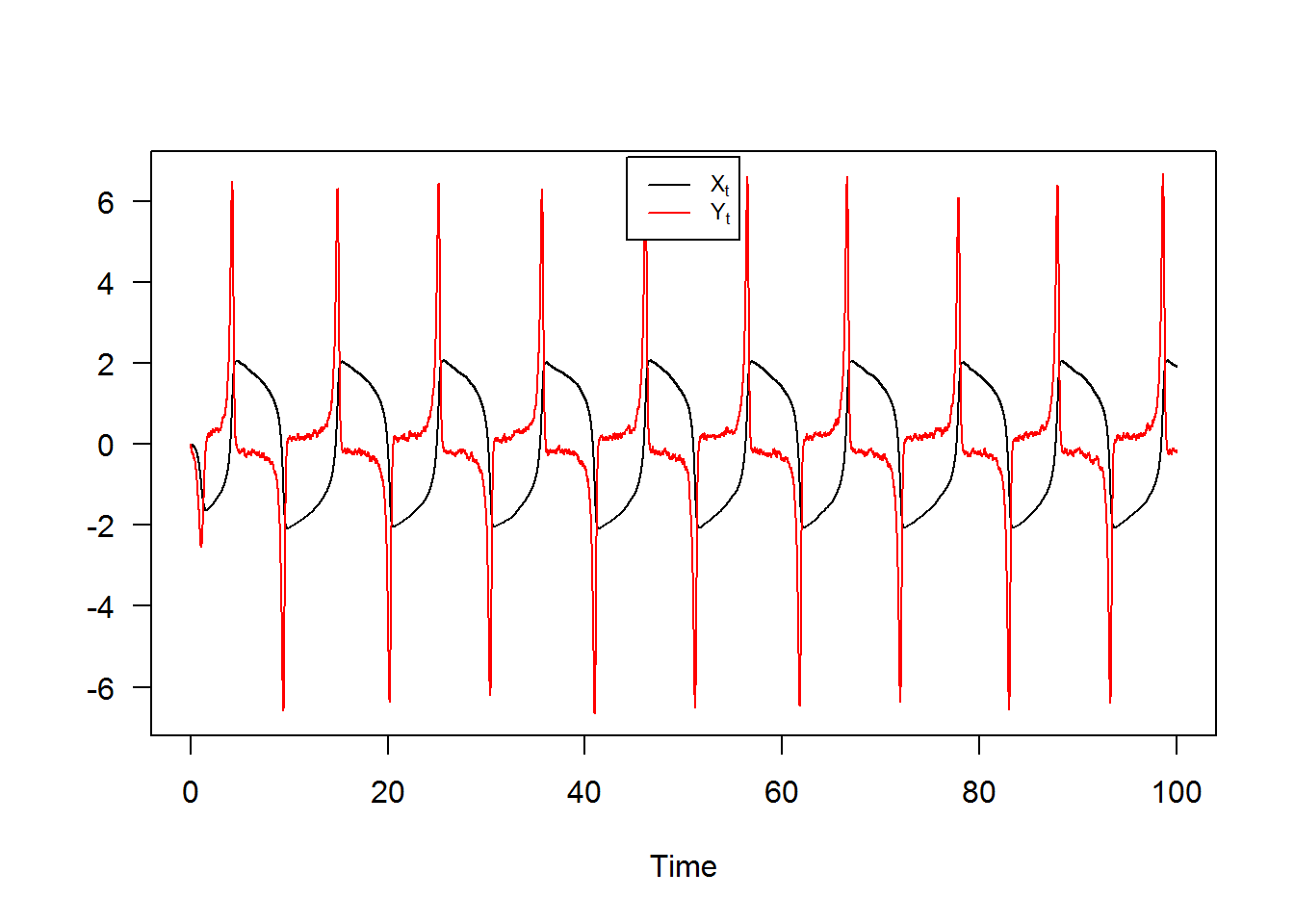Return to snssde2d()

# snssde3d()

The following $$3$$-dimensional SDE’s with a vector of drift and a diagonal matrix of diffusion coefficients:

It? form: $\begin{equation}\label{eq17} \begin{cases} dX_t = f_{x}(t,X_{t},Y_{t},Z_{t}) dt + g_{x}(t,X_{t},Y_{t},Z_{t}) dW_{1,t}\\ dY_t = f_{y}(t,X_{t},Y_{t},Z_{t}) dt + g_{y}(t,X_{t},Y_{t},Z_{t}) dW_{2,t}\\ dZ_t = f_{z}(t,X_{t},Y_{t},Z_{t}) dt + g_{z}(t,X_{t},Y_{t},Z_{t}) dW_{3,t} \end{cases} \end{equation}$

Stratonovich form: $\begin{equation}\label{eq18} \begin{cases} dX_t = f_{x}(t,X_{t},Y_{t},Z_{t}) dt + g_{x}(t,X_{t},Y_{t},Z_{t}) \circ dW_{1,t}\\ dY_t = f_{y}(t,X_{t},Y_{t},Z_{t}) dt + g_{y}(t,X_{t},Y_{t},Z_{t}) \circ dW_{2,t}\\ dZ_t = f_{z}(t,X_{t},Y_{t},Z_{t}) dt + g_{z}(t,X_{t},Y_{t},Z_{t}) \circ dW_{3,t} \end{cases} \end{equation}$ $$W_{1,t}$$, $$W_{2,t}$$ and $$W_{3,t}$$ is a 3 independent standard Wiener process. To simulate this system using snssde3d() function we need to specify:

• The drift (3d) and diffusion (3d) coefficients as R expressions that depend on the state variables x, y , z and time variable t.
• The number of simulation steps N (default: N=1000).
• The number of the solution trajectories to be simulated by M (default: M=1).
• The initial conditions t0, x0 and end time T (default: t0=0, x0=c(0,0,0) and T=1).
• The integration step size Dt (default: Dt=(T-t0)/N).
• The choice of process types by the argument type="ito" for Ito or type="str" for Stratonovich (default type="ito").
• The numerical method to be used by method (default method="euler").

## Basic example

Assume that we want to describe the following SDE’s (3D) in It? form: $\begin{equation}\label{eq0166} \begin{cases} dX_t = 4 (-1-X_{t}) Y_{t} dt + 0.2 dW_{1,t}\\ dY_t = 4 (1-Y_{t}) X_{t} dt + 0.2 dW_{2,t}\\ dZ_t = 4 (1-Z_{t}) Y_{t} dt + 0.2 dW_{3,t} \end{cases} \end{equation}$ We simulate a flow of $$10000$$ trajectories, with integration step size $$\Delta t = 0.001$$.

R> fx <- expression(4*(-1-x)*y , 4*(1-y)*x , 4*(1-z)*y)
R> gx <- rep(expression(0.2),3)
R> mod3d <- snssde3d(x0=c(x=2,y=-2,z=-2),drift=fx,diffusion=gx,M=1000)
R> mod3d
Itô Sde 3D:
| dX(t) = 4 * (-1 - X(t)) * Y(t) * dt + 0.2 * dW1(t)
| dY(t) = 4 * (1 - Y(t)) * X(t) * dt + 0.2 * dW2(t)
| dZ(t) = 4 * (1 - Z(t)) * Y(t) * dt + 0.2 * dW3(t)
Method:
| Euler scheme with order 0.5
Summary:
| Size of process   | N  = 1001.
| Number of simulation  | M  = 1000.
| Initial values    | (x0,y0,z0) = (2,-2,-2).
| Time of process   | t in [0,1].
| Discretization    | Dt = 0.001.

The following statistical measures (S3 method) for class snssde3d() can be approximated for the $$(X_{t},Y_{t},Z_{t})$$ process at any time $$t$$, for example at=1:

R> s = 1
R> mean(mod3d, at = s)
 -0.79771  0.87700  0.79586
R> moment(mod3d, at = s , center = TRUE , order = 2) ## variance
 0.0098837 0.1097268 0.0099780
R> Median(mod3d, at = s)
 -0.79993  0.83984  0.80182
R> Mode(mod3d, at = s)
 -0.78944  0.76454  0.80311
R> quantile(mod3d , at = s)
$x 0% 25% 50% 75% 100% -1.08274 -0.86567 -0.79993 -0.73760 -0.35725$y
0%      25%      50%      75%     100%
0.057898 0.644261 0.839842 1.073442 2.203195

$z 0% 25% 50% 75% 100% 0.38141 0.73503 0.80182 0.86654 1.07719  R> kurtosis(mod3d , at = s)  3.3793 3.5112 3.7198 R> skewness(mod3d , at = s)  0.32230 0.54207 -0.52200 R> cv(mod3d , at = s )  -0.12469 0.37790 0.12557 R> min(mod3d , at = s)  -1.082739 0.057898 0.381408 R> max(mod3d , at = s)  -0.35725 2.20320 1.07719 R> moment(mod3d, at = s , center= TRUE , order = 4)  0.00033078 0.04236003 0.00037109 R> moment(mod3d, at = s , center= FALSE , order = 4)  0.44198 1.20952 0.43782 The summary of the results of mod3d at time $$t=1$$ of class snssde3d() is given by: R> summary(mod3d, at = s)  Monte-Carlo Statistics for (X(t),Y(t),Z(t)) at time t = 1 X Y Z Mean -0.79771 0.87700 0.79586 Variance 0.00989 0.10984 0.00999 Median -0.79993 0.83984 0.80182 Mode -0.78944 0.76454 0.80311 First quartile -0.86567 0.64426 0.73503 Third quartile -0.73760 1.07344 0.86654 Minimum -1.08274 0.05790 0.38141 Maximum -0.35725 2.20320 1.07719 Skewness 0.32230 0.54207 -0.52200 Kurtosis 3.37935 3.51125 3.71979 Coef-variation -0.12469 0.37790 0.12557 3th-order moment 0.00032 0.01973 -0.00052 4th-order moment 0.00033 0.04236 0.00037 5th-order moment 0.00004 0.02313 -0.00006 6th-order moment 0.00002 0.03233 0.00003 For plotting (back in time) using the command plot, and plot3D in space the results of the simulation are shown in Figure 9. R> plot(mod3d,union = TRUE) ## back in time R> plot3D(mod3d,display="persp") ## in space (O,X,Y,Z)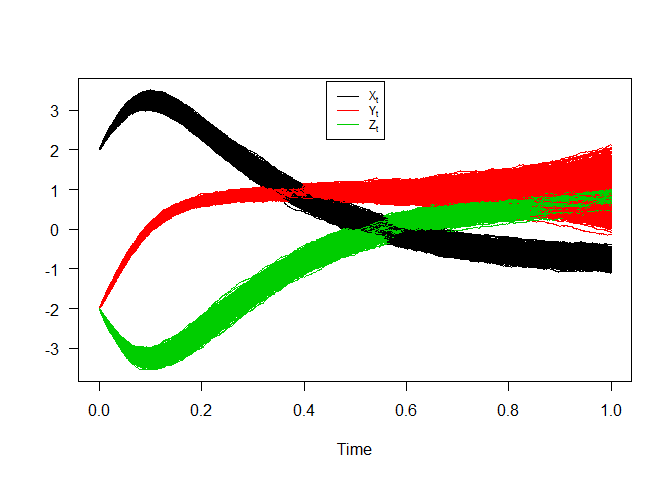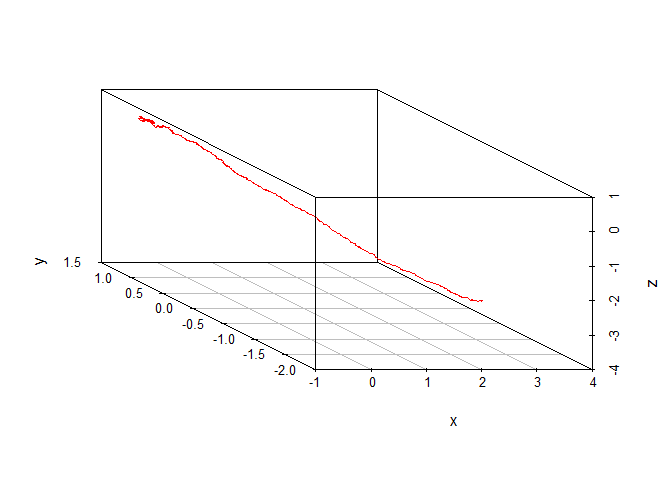3D SDEs Hence we can just make use of the rsde3d() function to build our random number for $$(X_{t},Y_{t},Z_{t})$$ at time $$t = 1$$. R> out <- rsde3d(object = mod3d, at = s) R> head(out,n=10)  x y z 1 -0.56659 0.40010 0.58340 2 -0.83126 0.54093 0.65433 3 -0.96898 1.30182 0.82791 4 -0.85467 0.91820 0.82323 5 -0.74002 0.85950 0.72057 6 -0.72922 0.84603 0.88307 7 -0.68068 0.56023 0.57196 8 -0.77963 0.62726 0.75782 9 -0.61304 0.54800 0.72629 10 -0.86653 1.26019 0.94123 R> summary(out)  x y z Min. :-1.083 Min. :0.0579 Min. :0.381 1st Qu.:-0.866 1st Qu.:0.6443 1st Qu.:0.735 Median :-0.800 Median :0.8398 Median :0.802 Mean :-0.798 Mean :0.8770 Mean :0.796 3rd Qu.:-0.738 3rd Qu.:1.0734 3rd Qu.:0.867 Max. :-0.357 Max. :2.2032 Max. :1.077  R> cov(out)  x y z x 0.0098936 -0.018792 -0.0043937 y -0.0187917 0.109837 0.0200126 z -0.0043937 0.020013 0.0099880 For each SDE type and for each numerical scheme, the marginal density of $$X_t$$, $$Y_t$$ and $$Z_t$$ at time $$t=1$$ are reported using dsde3d() function, see e.g. Figure 10. R> den <- dsde3d(mod3d,pdf="M",at =1) R> den  Marginal density of X(t-t0)|X(t0)=2 at time t = 1 Data: x (1000 obs.); Bandwidth 'bw' = 0.02161 x f(x) Min. :-1.14756 Min. :0.0002 1st Qu.:-0.93378 1st Qu.:0.0279 Median :-0.72000 Median :0.4314 Mean :-0.72000 Mean :1.1683 3rd Qu.:-0.50621 3rd Qu.:2.1636 Max. :-0.29243 Max. :4.0682 Marginal density of Y(t-t0)|Y(t0)=-2 at time t = 1 Data: y (1000 obs.); Bandwidth 'bw' = 0.07241 y f(y) Min. :-0.15932 Min. :0.00006 1st Qu.: 0.48561 1st Qu.:0.02870 Median : 1.13055 Median :0.17131 Mean : 1.13055 Mean :0.38726 3rd Qu.: 1.77548 3rd Qu.:0.75625 Max. : 2.42042 Max. :1.23074 Marginal density of Z(t-t0)|Z(t0)=-2 at time t = 1 Data: z (1000 obs.); Bandwidth 'bw' = 0.02219 z f(z) Min. :0.31485 Min. :0.0002 1st Qu.:0.52207 1st Qu.:0.0567 Median :0.72930 Median :0.4489 Mean :0.72930 Mean :1.2052 3rd Qu.:0.93653 3rd Qu.:2.3340 Max. :1.14375 Max. :4.2142  R> plot(den, main="Marginal Density")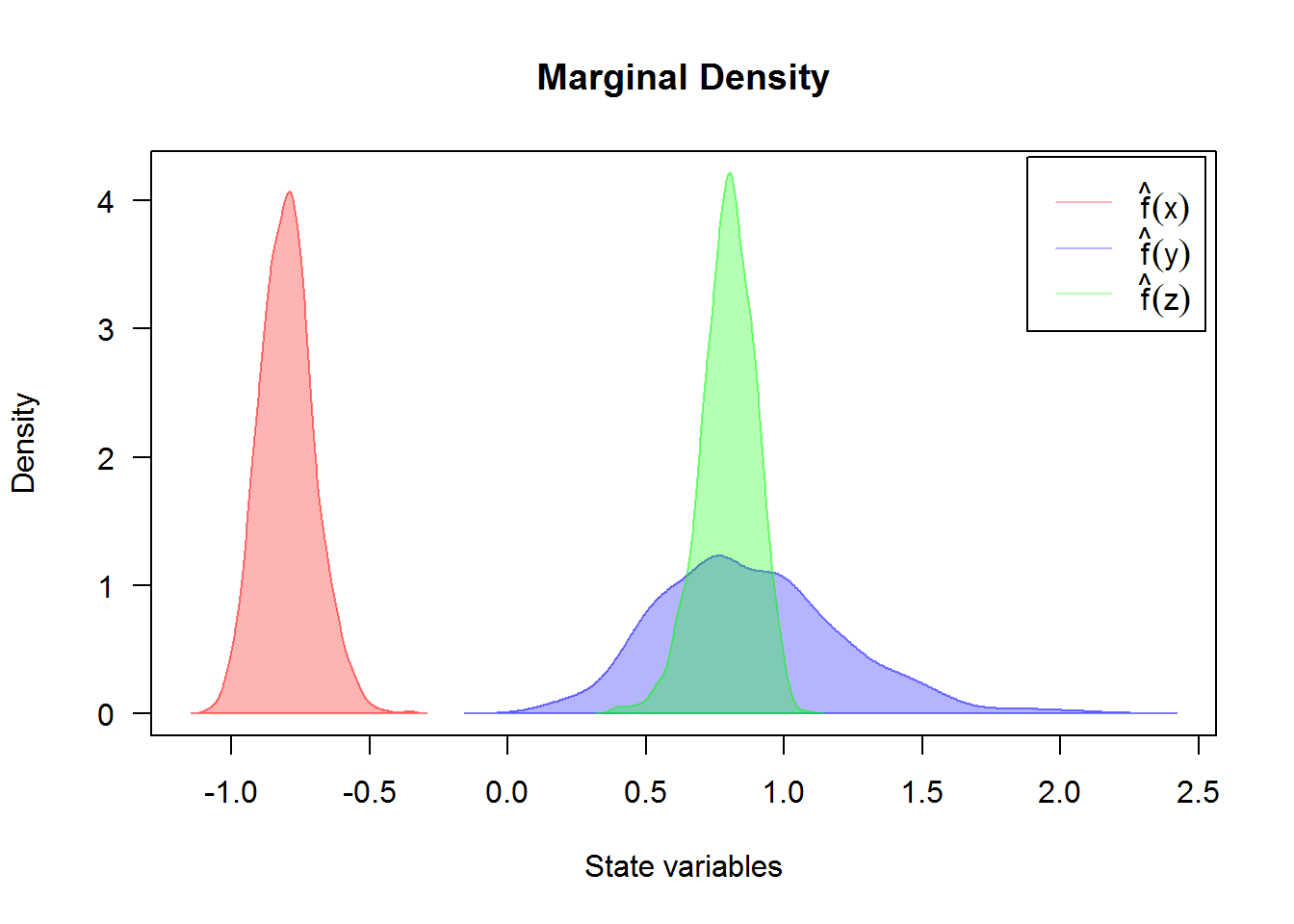For an approximate joint transition density for $$(X_t,Y_t,Z_t)$$ (for more details, see package sm or ks.) R> denJ <- dsde3d(mod3d,pdf="J") R> plot(denJ,display="rgl") Return to snssde3d() ## Attractive model for 3D diffusion processes If we assume that $$U_w( x , y , z , t )$$, $$V_w( x , y , z , t )$$ and $$S_w( x , y , z , t )$$ are neglected and the dispersion coefficient $$D( x , y , z )$$ is constant. A system becomes (see Boukhetala,1996): $\begin{eqnarray}\label{eq19} % \nonumber to remove numbering (before each equation) \begin{cases} dX_t = \left(\frac{-K X_{t}}{\sqrt{X^{2}_{t} + Y^{2}_{t} + Z^{2}_{t}}}\right) dt + \sigma dW_{1,t} \nonumber\\ dY_t = \left(\frac{-K Y_{t}}{\sqrt{X^{2}_{t} + Y^{2}_{t} + Z^{2}_{t}}}\right) dt + \sigma dW_{2,t} \\ dZ_t = \left(\frac{-K Z_{t}}{\sqrt{X^{2}_{t} + Y^{2}_{t} + Z^{2}_{t}}}\right) dt + \sigma dW_{3,t} \nonumber \end{cases} \end{eqnarray}$ with initial conditions $$(X_{0},Y_{0},Z_{0})=(1,1,1)$$, by specifying the drift and diffusion coefficients of three processes $$X_{t}$$, $$Y_{t}$$ and $$Z_{t}$$ as R expressions which depends on the three state variables (x,y,z) and time variable t, with integration step size Dt=0.0001. R> K = 4; s = 1; sigma = 0.2 R> fx <- expression( (-K*x/sqrt(x^2+y^2+z^2)) , (-K*y/sqrt(x^2+y^2+z^2)) , (-K*z/sqrt(x^2+y^2+z^2)) ) R> gx <- rep(expression(sigma),3) R> mod3d <- snssde3d(drift=fx,diffusion=gx,N=10000,x0=c(x=1,y=1,z=1)) R> mod3d Itô Sde 3D: | dX(t) = (-K * X(t)/sqrt(X(t)^2 + Y(t)^2 + Z(t)^2)) * dt + sigma * dW1(t) | dY(t) = (-K * Y(t)/sqrt(X(t)^2 + Y(t)^2 + Z(t)^2)) * dt + sigma * dW2(t) | dZ(t) = (-K * Z(t)/sqrt(X(t)^2 + Y(t)^2 + Z(t)^2)) * dt + sigma * dW3(t) Method: | Euler scheme with order 0.5 Summary: | Size of process | N = 10001. | Number of simulation | M = 1. | Initial values | (x0,y0,z0) = (1,1,1). | Time of process | t in [0,1]. | Discretization | Dt = 0.0001. The results of simulation are shown: R> plot3D(mod3d,display="persp",col="blue")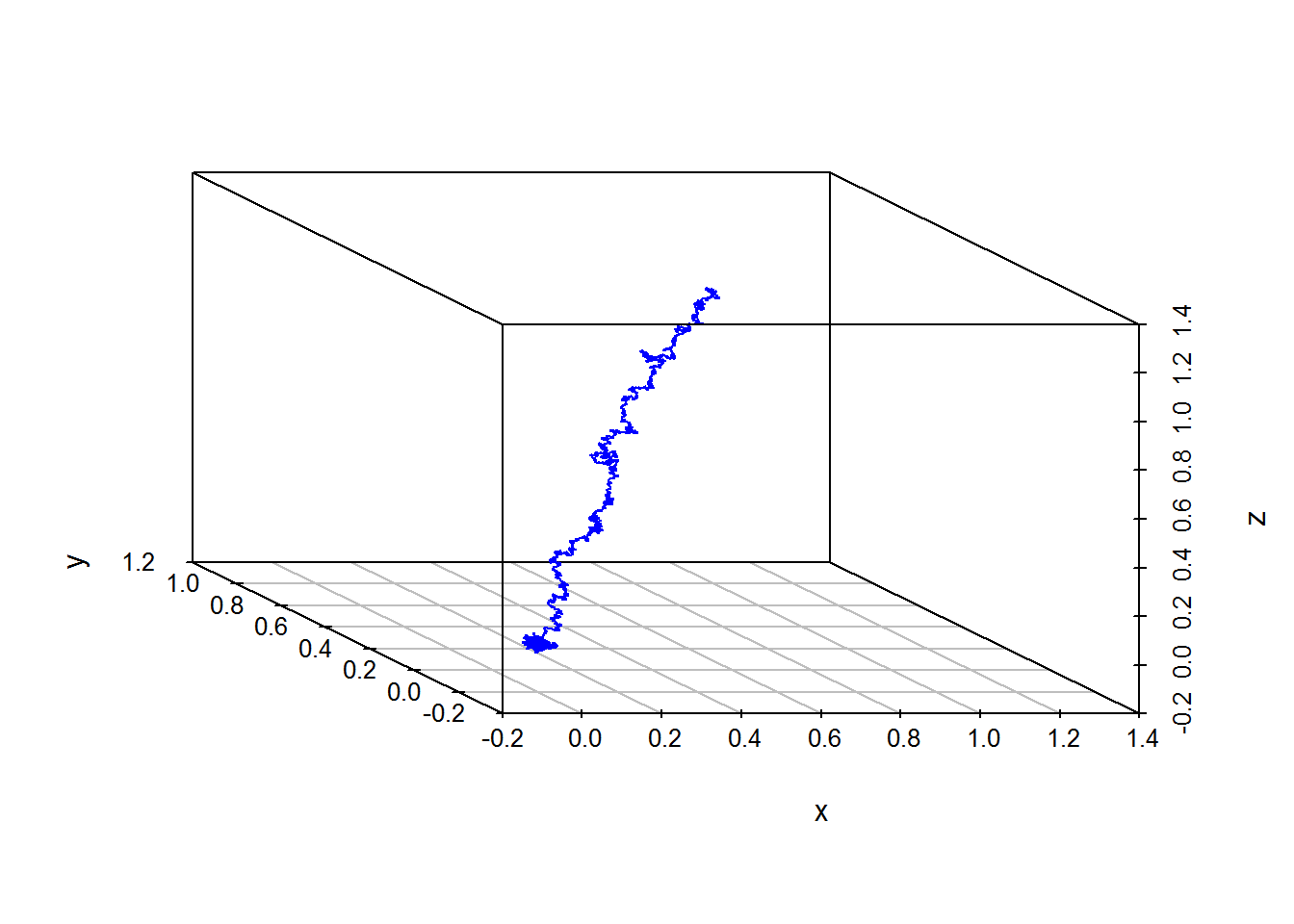Return to snssde3d() ## Transformation of an SDE one-dimensional Next is an example of one-dimensional SDE driven by three independent Brownian motions ($$W_{1,t}$$,$$W_{2,t}$$,$$W_{3,t}$$), as follows: $\begin{equation}\label{eq20} dX_{t} = \mu W_{1,t} dt + \sigma W_{2,t} \circ dW_{3,t} \end{equation}$ To simulate the solution of the process $$X_t$$, we make a transformation to a system of three equations as follows: $\begin{eqnarray}\label{eq21} \begin{cases} % \nonumber to remove numbering (before each equation) dX_t = \mu Y_{t} dt + \sigma Z_{t} \circ dW_{3,t} \nonumber\\ dY_t = dW_{1,t} \\ dZ_t = dW_{2,t} \nonumber \end{cases} \end{eqnarray}$ run by calling the function snssde3d() to produce a simulation of the solution, with $$\mu = 1$$ and $$\sigma = 1$$. R> fx <- expression(y,0,0) R> gx <- expression(z,1,1) R> modtra <- snssde3d(drift=fx,diffusion=gx,M=1000,type="str") R> modtra Stratonovich Sde 3D: | dX(t) = Y(t) * dt + Z(t) o dW1(t) | dY(t) = 0 * dt + 1 o dW2(t) | dZ(t) = 0 * dt + 1 o dW3(t) Method: | Euler scheme with order 0.5 Summary: | Size of process | N = 1001. | Number of simulation | M = 1000. | Initial values | (x0,y0,z0) = (0,0,0). | Time of process | t in [0,1]. | Discretization | Dt = 0.001. R> summary(modtra)  Monte-Carlo Statistics for (X(t),Y(t),Z(t)) at time t = 1 X Y Z Mean 0.02050 -0.00735 0.00410 Variance 0.76290 1.02520 0.92697 Median -0.00513 0.00347 -0.00932 Mode 0.08659 -0.10735 -0.07669 First quartile -0.50274 -0.69009 -0.63180 Third quartile 0.52399 0.69699 0.66827 Minimum -2.80265 -3.26565 -3.90726 Maximum 5.99953 3.72179 3.17907 Skewness 0.46773 -0.03733 -0.12839 Kurtosis 5.74922 3.15054 3.41486 Coef-variation 42.61668 -137.73620 234.60715 3th-order moment 0.31167 -0.03875 -0.11459 4th-order moment 3.34617 3.31130 2.93428 5th-order moment 8.66966 0.11897 -1.59067 6th-order moment 56.80855 18.73499 18.05038 the following code produces the result in Figure 12. R> plot(modtra$X,plot.type="single",ylab=expression(X[t]))
R> lines(time(modtra),apply(modtra$X,1,mean),col=2,lwd=2) R> legend("topleft",c("mean path"),col=2,lwd=2,cex=0.8)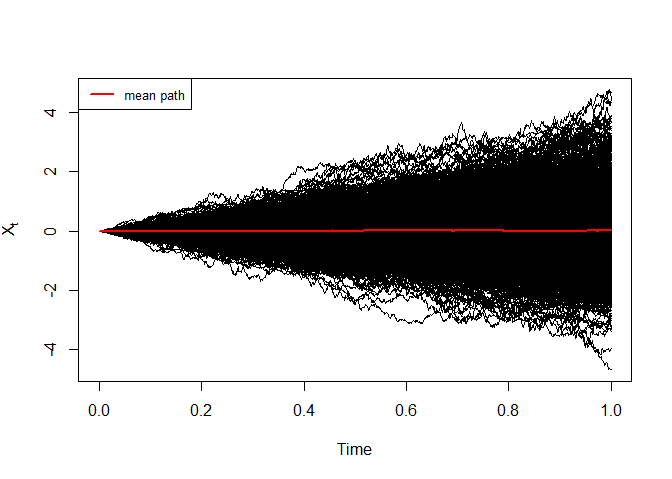Simulation of $$X_t$$ The histogram and kernel density of $$X_t$$ at time $$t=1$$ are reported using dsde3d() function, see e.g. Figure 13. R> den <- dsde3d(modtra,pdf="Marginal",at=1) R> den$resx

Call:
density.default(x = x, na.rm = TRUE)

Data: x (1000 obs.);    Bandwidth 'bw' = 0.1732

x                y
Min.   :-3.322   Min.   :0.00000
1st Qu.:-0.862   1st Qu.:0.00058
Median : 1.598   Median :0.01768
Mean   : 1.598   Mean   :0.10151
3rd Qu.: 4.059   3rd Qu.:0.14044
Max.   : 6.519   Max.   :0.50564  
R> MASS::truehist(den$ech$x,xlab = expression(X[t==1]));box()
R> lines(den$resx,col="red",lwd=2) R> legend("topleft",c("Distribution histogram","Kernel Density"),inset =.01,pch=c(15,NA),lty=c(NA,1),col=c("cyan","red"), lwd=2,cex=0.8)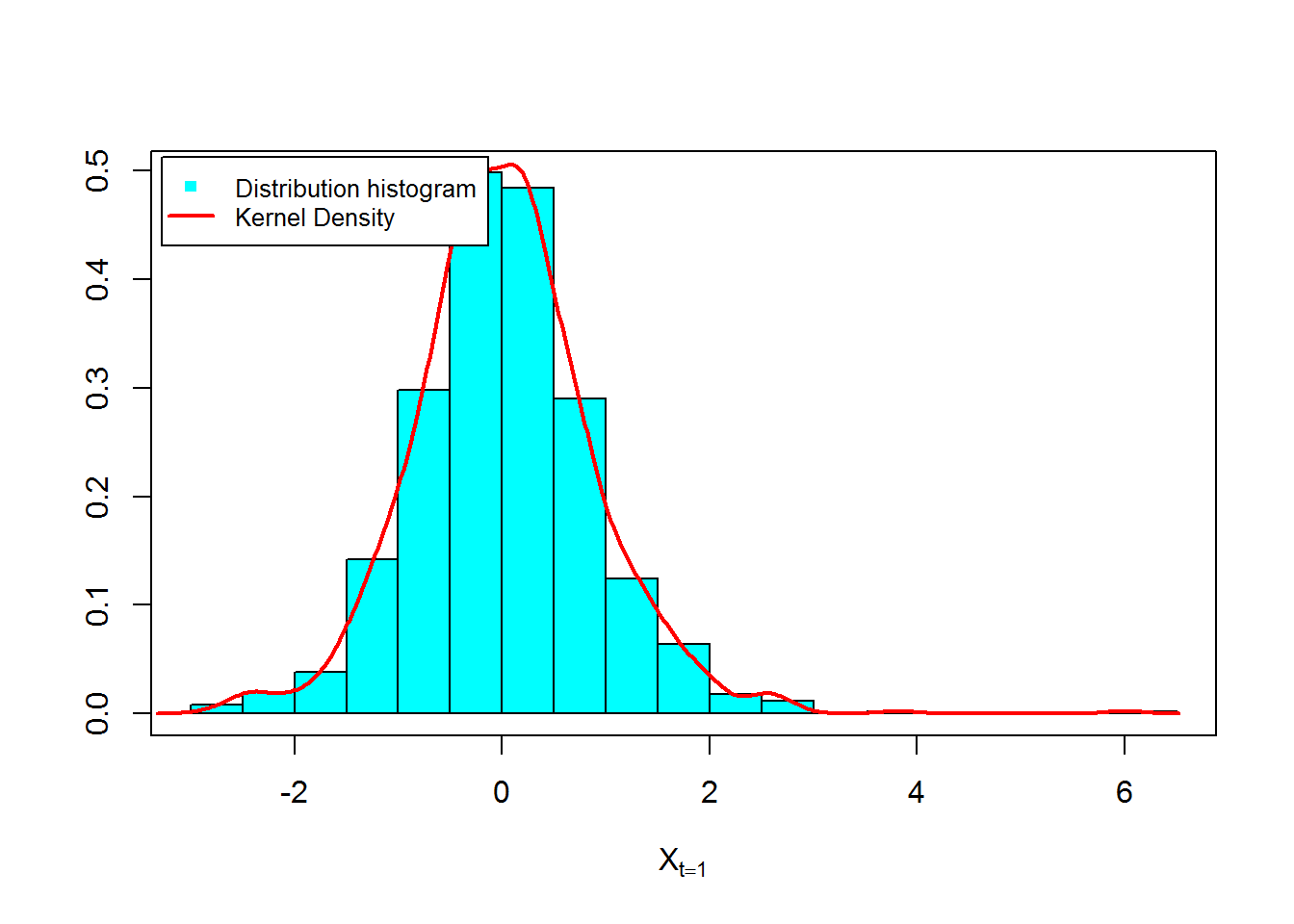Figure 14 and 15, show approximation results for $$m_{1}(t)= \text{E}(X_{t})$$, $$S_{1}(t)=\text{V}(X_{t})$$ and $$C(s,t)=\text{Cov}(X_{s},X_{t})$$: R> m1 <- apply(modtra$X,1,mean) ## m1(t)
R> S1  <- apply(modtra$X,1,var) ## s1(t) R> out_a <- data.frame(m1,S1) R> matplot(time(modtra), out_a, type = "l", xlab = "time", ylab = "", col=2:3,lwd=2,lty=2:3,las=1) R> legend("topleft",c(expression(m(t),S(t))),inset = .09,col=2:3,lty=2:3,lwd=2,cex=0.9)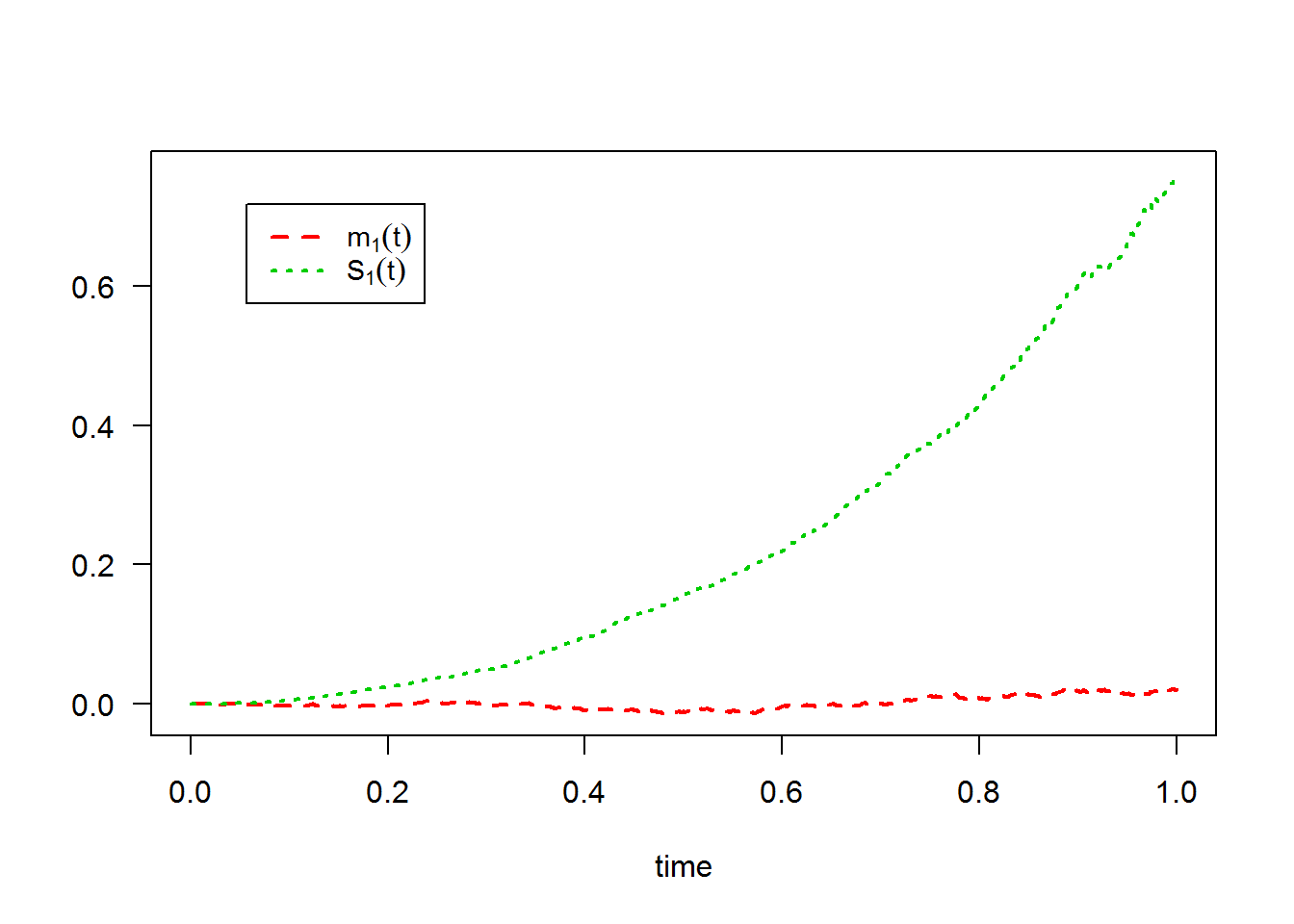R> color.palette=colorRampPalette(c('white','green','blue','red')) R> filled.contour(time(modtra), time(modtra), cov(t(modtra$X)), color.palette=color.palette,plot.title = title(main = expression(paste("Covariance empirique:",cov(X[s],X[t]))),xlab = "time", ylab = "time"),key.title = title(main = ""))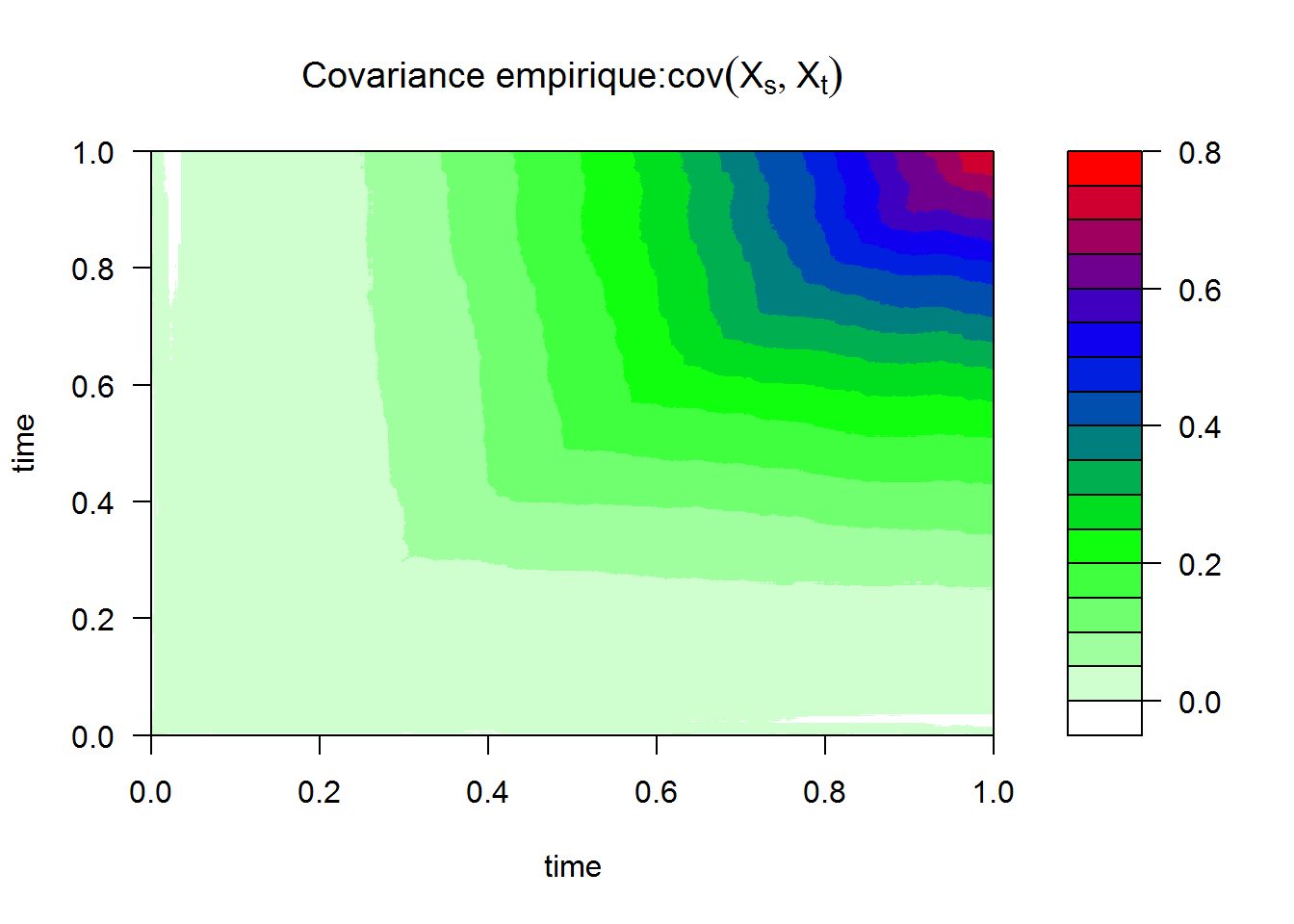Return to snssde3d()

1. Department of Probabilities & Statistics, Faculty of Mathematics, University of Science and Technology Houari Boumediene, BP 32 El-Alia, U.S.T.H.B, Algeria, E-mail ()

2. Faculty of Mathematics, University of Science and Technology Houari Boumediene, BP 32 El-Alia, U.S.T.H.B, Algeria, E-mail ()

3. The equivalently of $$X_{t}^{\text{mod1}}$$ the following Stratonovich SDE: $$dX_{t} = \theta X_{t} \circ dW_{t}$$.